# C++ 并发与多线程学习笔记（三）线程传参隐患 成员函数指针做线程函数

## 传递临时对象作为线程参数

#include <string>
#include <iostream>
using namespace std;
void myprint(const int &i,char *pmybuf)
{
cout << i << endl;
cout << pmybuf << endl;
return;
}

int main()
{
int mvar = 1;
int &mvary = mvar;
char mybuf[] = "this is a test!";
mytobj.detach();
cout << "主线程结束" << endl;
system("pause");
return 0;
}


（我用的是x64）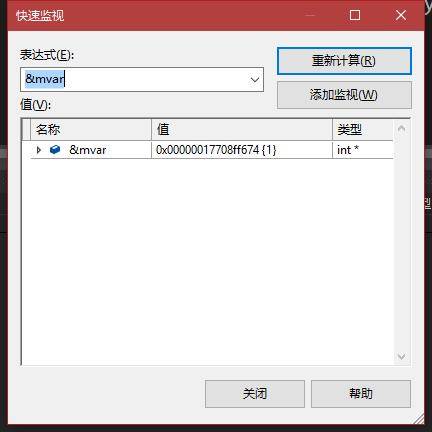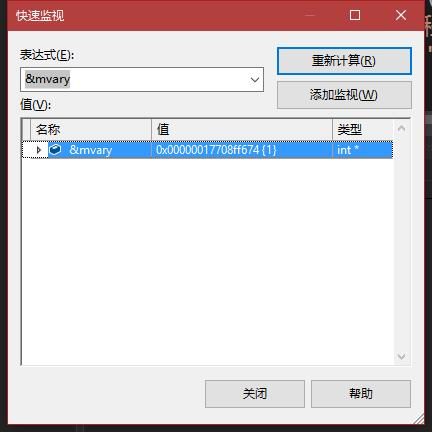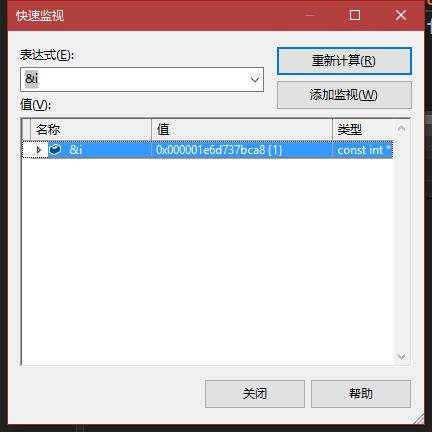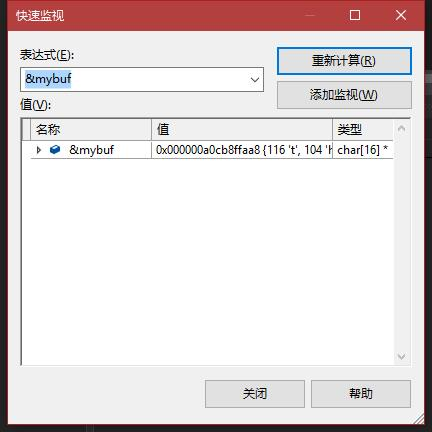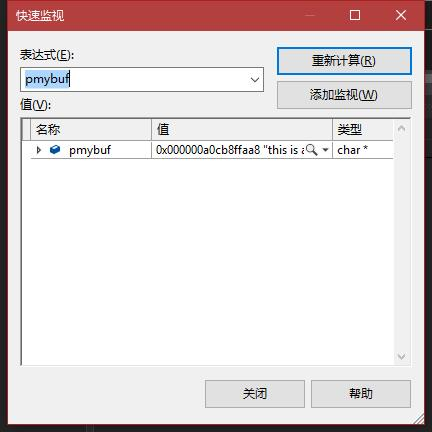void myprint(const int &i,const std::string &pmybuf)
{
cout << i << endl;
cout << pmybuf << endl;
return;
}


thread mytobj(myprint,mvar,std::string(mybuf));


#include <string>
#include <iostream>
using namespace std;
class A
{
public:
int m_i;
A(int a) :m_i(a)
{
cout << "A:A(int a)构造函数执行" << endl;
}
A(const A &a) :m_i(a.m_i)
{
cout << "A:A(const A &a)构造函数执行" << endl;
}
~A()
{
cout << "析构函数执行" << endl;
}
};

void myprint(const int &i, const A &pmybuf)
{
cout << i << endl;
cout << &pmybuf << endl;	//打印地址
return;
}

int main()
{
int mvar = 1;
int mysecondpar = 12;
//将mysecond转换成A类对象传递给myprint的第二个参数
mytobj.join();
//mytobj.detach();
cout << "主线程结束" << endl;
system("pause");
return 0;
}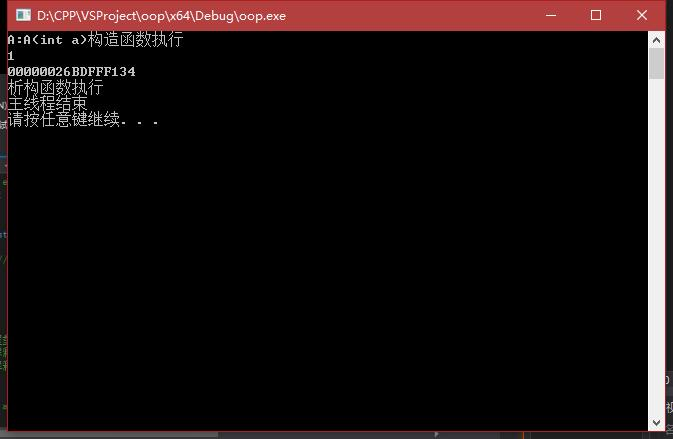mytobj.join();
//mytobj.detach();

//更改为

//mytobj.join();
mytobj.detach();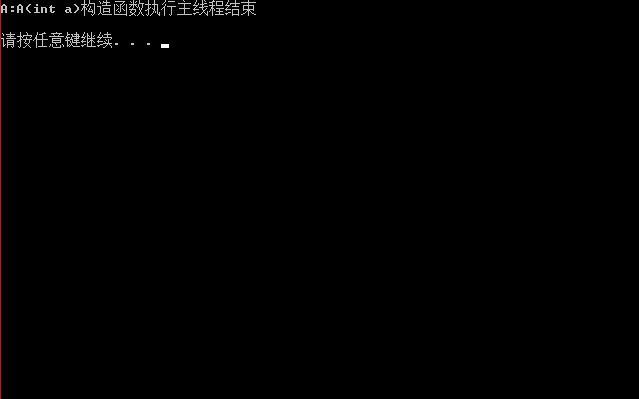thread mytobj(myprint, mvar, mysecondpar);
//更改为：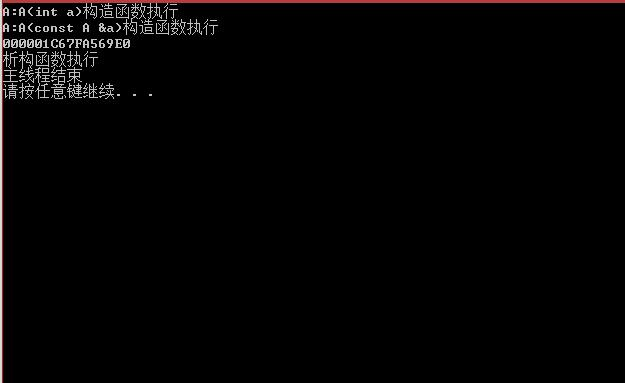1. 若传递int这种简单类型参数，建议使用值传递，不要使用引用和指针，防止节外生枝。
2. 如果传递类对象，避免使用隐式类型转换，可以使用构建临时对象，在函数参数中使用引用可以少使用一次构造函数（避免浪费空间）
3. 建议不使用detach()，只使用join()，这样不存在局部变量失效对内存非法引用的问题。

## 线程ID

#include <string>
#include <iostream>
using namespace std;
class A
{
public:
int m_i;
A(int a) :m_i(a)
{
cout << "A:A(int a)构造函数执行"  << this_thread::get_id() << endl;
}
A(const A &a) :m_i(a.m_i)
{
cout << "A:A(const A &a)构造函数执行" << this_thread::get_id() << endl;
}
~A()
{
cout << "析构函数执行" << this_thread::get_id() << endl;
}
};

void myprint(const A &pmybuf)
{
cout << &pmybuf << this_thread::get_id() << endl;	//打印地址
return;
}

int main()
{
int mvar = 1;
int mysecondpar = 12;
//将mysecond转换成A类对象传递给myprint的第二个参数
//mytobj.join();
mytobj.detach();

cout << "主线程结束" << endl;
//system("pause");
return 0;
}


## 传递类对象、智能指针作为线程参数

std::ref函数

A myobj(10);


### 使用智能指针

void myprint(unique_ptr<int> pzn)
{
cout << &pzn << this_thread::get_id() << endl;	//打印地址
return;
}

int main()
{
int mvar = 1;
int mysecondpar = 12;

unique_ptr<int> myp(new int(100));

mytobj.join();
//mytobj.detach();

cout << "主线程结束" << endl;
//system("pause");
return 0;
}


## 用成员函数指针做线程函数

#include <string>
#include <iostream>
using namespace std;
class A
{
public:
int m_i;
A(int a) :m_i(a)
{
cout << "A:A(int a)构造函数执行"  << " ,id: " << this_thread::get_id() << endl;
}
A(const A &a) :m_i(a.m_i)
{
cout << "A:A(const A &a)构造函数执行" << " ,id: " << this_thread::get_id() << endl;
}
~A()
{
cout << "析构函数执行" << " ,id: " << this_thread::get_id() << endl;
}

{
cout << "thread_work执行了" << n << " ,id: " << this_thread::get_id() << endl;
}
};

int main()
{
int mvar = 1;
int mysecondpar = 12;

A a(10);

mytobj.join();
//mytobj.detach();

cout << "主线程结束" << endl;
//system("pause");
return 0;
}


thread mytobj(&A::thread_work, a,10);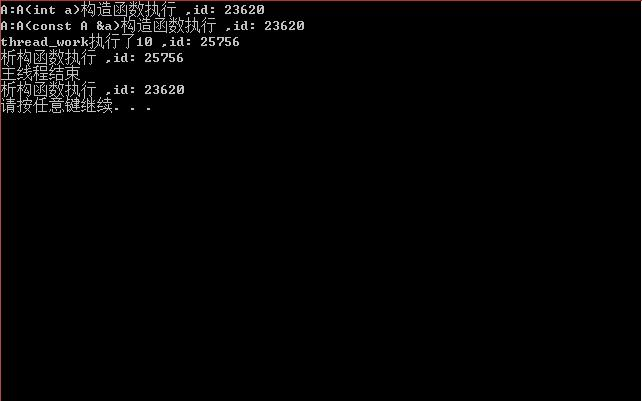01-31694202-15687
06-11227
12-031万+
01-201万+
03-01192
11-01115
02-2386
03-1636
02-21137
02-16194
08-3054
09-2813
03-0245
01-053077
06-111372
10-1876
01-041005
09-03531
09-1449